Pauli matrices

Certain special constant Hermitian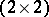-matrices with complex entries. They were introduced by W. Pauli (1927) to describe spin (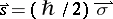) and magnetic moment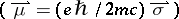of an electron. His equation describes correctly in the non-relativistic case particles of spin 1/2 (in units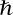) and can be obtained from the Dirac equation for. In explicit form the Pauli matrices are: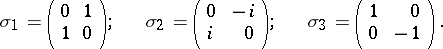Their eigen values are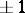. The Pauli matrices satisfy the following algebraic relations: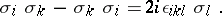Together with the unit matrixthe Pauli matrices form a complete system of second-order matrices by which an arbitrary linear operator (matrix) of dimension 2 can be expanded. They act on two-component spin functions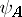,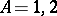, and are transformed under a rotation of the coordinate system by a linear two-valued representation of the rotation group. Under a rotation by an infinitesimal anglearound an axis with a directed unit vector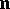, a spinoris transformed according to the formulaFrom the Pauli matrices one can form the Dirac matrices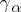,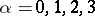:The real linear combinations of,,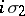,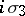form a four-dimensional subalgebra of the algebra of complex-matrices (under matrix multiplication) that is isomorphic to the simplest system of hypercomplex numbers, the quaternions, cf. Quaternion. They are used whenever an elementary particle has a discrete parameter taking only two values, for example, to describe an isospin nucleon (a proton-neutron). Quite generally, the Pauli matrices are used not only to describe isotopic space, but also in the formalism of the group of inner symmetries. In this case they are generators of a-dimensional representation of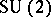and are denoted by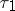,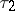and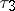. Sometimes it is convenient to use the linear combinations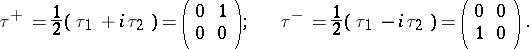In certain cases one introduces for a relativistically covariant description of two-component spinor functions instead of the Pauli matrices, matricesrelated by means of the following identities: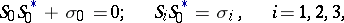(1)

where the symboldenotes complex conjugation. The matricessatisfy the commutator relations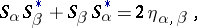(2)

where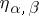are the components of the metric tensor of the Minkowski space of signature. The formulas (1) and (2) make it possible to generalize the Pauli matrices covariantly to an arbitrary curved space: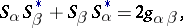where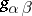are the components of the metric tensor of the curved space.